# A glass cpillary sealed at the upper end is of length 0.11 m and internal diameter 0.02 mm . The tube is immersed vertically into a liquid of surface tension 0.0506 N/m.To what length has the capillary to be immersed so that the liquid levels inside and outside the capillary become the same ? What will happen to the water levels inside the capillary if the seal is now broken ?

8 years ago
p { margin-bottom: 0.25cm; line-height: 120%; }

Look at the picture. When liquid levels are same inside and outside of the tube.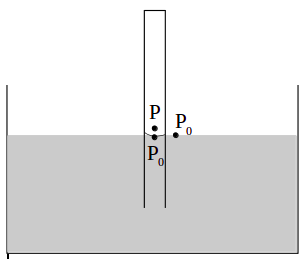p { margin-bottom: 0.25cm; line-height: 120%; }

Let L is the total length of the tube and y is the portion immersed.

T = surface tension and r is the radius of the tube.

Po = atmospheric pressure and P = pressure inside the tube above liquid level

Air pressure in the tube is given by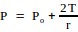Initially when the tube was not immersed, the air pressure inside the tube was Po.

From ideal gas equation assuming same temperature before and after immersion,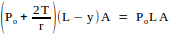Where A = area of cross section of the tube.

Solving for y, we get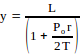Substituting the values, we get y = .01m = 1 cm.

Now when the seal is broken, the liquid will rise to a height h inside the tube given

by the formula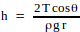, where θ is the angle of contact and ρ is the density of

the liquid.